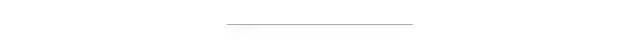# 面试：BigDecimal一定不会丢失精度吗？``System.out.println(0.05 + 0.01);  System.out.println(1.0 - 0.42);  System.out.println(4.015 * 100);  System.out.println(123.3 / 100);  ``

0.060000000000000005
0.5800000000000001
401.49999999999994
1.2329999999999999

Java中float的精度为6-7位有效数字。double的精度为15-16位。

#### API

``构造器                   描述BigDecimal(int)       创建一个具有参数所指定整数值的对象。BigDecimal(double)    创建一个具有参数所指定双精度值的对象。BigDecimal(long)      创建一个具有参数所指定长整数值的对象。BigDecimal(String)    创建一个具有参数所指定以字符串表示的数值的对象。``

``方法                    描述add(BigDecimal)       BigDecimal对象中的值相加，然后返回这个对象。subtract(BigDecimal)  BigDecimal对象中的值相减，然后返回这个对象。multiply(BigDecimal)  BigDecimal对象中的值相乘，然后返回这个对象。divide(BigDecimal)    BigDecimal对象中的值相除，然后返回这个对象。toString()            将BigDecimal对象的数值转换成字符串。doubleValue()         将BigDecimal对象中的值以双精度数返回。floatValue()          将BigDecimal对象中的值以单精度数返回。longValue()           将BigDecimal对象中的值以长整数返回。intValue()            将BigDecimal对象中的值以整数返回。``

### BigDecimal精度也丢失

``BigDecimal a = new BigDecimal(1.01);BigDecimal b = new BigDecimal(1.02);BigDecimal c = new BigDecimal("1.01");BigDecimal d = new BigDecimal("1.02");System.out.println(a.add(b));System.out.println(c.add(d));``

2.0300000000000000266453525910037569701671600341796875
2.03

long可以准确存储19位数字，而double只能准备存储16位数字。

double由于有exp位，可以存16位以上的数字，但是需要以低位的不精确作为代价。如果需要高于19位数字的精确存储，则必须用BigInteger来保存，当然会牺牲一些性能。

``* The results of this constructor can be somewhat unpredictable.       * One might assume that writing {@codenew BigDecimal(0.1)} in       * Java creates a {@code BigDecimal} which is exactly equal to       * 0.1 (an unscaled value of 1, with a scale of 1), but it is       * actually equal to       * 0.1000000000000000055511151231257827021181583404541015625.       * This is because 0.1 cannot be represented exactly as a       * {@codedouble} (or, for that matter, as a binary fraction of       * any finite length).  Thus, the value that is being passed       * <i>in</i> to the constructor is not exactly equal to 0.1,       * appearances notwithstanding.         ……          * When a {@codedouble} must be used as a source for a       * {@code BigDecimal}, note that this constructor provides an       * exact conversion; it does not give the same result as       * converting the {@codedouble} to a {@code String} using the       * {@link Double#toString(double)} method and then using the       * {@link #BigDecimal(String)} constructor.  To get that result,       * use the {@codestatic} {@link #valueOf(double)} method.       * </ol>  public BigDecimal(double val) {      this(val,MathContext.UNLIMITED);  }  ``

### 正确运用BigDecimal

``/**   * @author: Ji YongGuang.   * @date: 19:50 2017/12/14.   */  publicclass BigDecimalUtil {      private BigDecimalUtil() {      }      public static BigDecimal add(double v1, double v2) {// v1 + v2          BigDecimal b1 = new BigDecimal(Double.toString(v1));          BigDecimal b2 = new BigDecimal(Double.toString(v2));          return b1.add(b2);      }      public static BigDecimal sub(double v1, double v2) {          BigDecimal b1 = new BigDecimal(Double.toString(v1));          BigDecimal b2 = new BigDecimal(Double.toString(v2));          return b1.subtract(b2);      }      public static BigDecimal mul(double v1, double v2) {          BigDecimal b1 = new BigDecimal(Double.toString(v1));          BigDecimal b2 = new BigDecimal(Double.toString(v2));          return b1.multiply(b2);      }      public static BigDecimal div(double v1, double v2) {          BigDecimal b1 = new BigDecimal(Double.toString(v1));          BigDecimal b2 = new BigDecimal(Double.toString(v2));          // 2 = 保留小数点后两位   ROUND_HALF_UP = 四舍五入          return b1.divide(b2, 2, BigDecimal.ROUND_HALF_UP);// 应对除不尽的情况      }  }  ``

Spring Boot 2.x基础教程：MyBatis的多数据源配置#### 已有 0 条评论

欢迎您，新朋友，感谢参与互动！
// 友盟